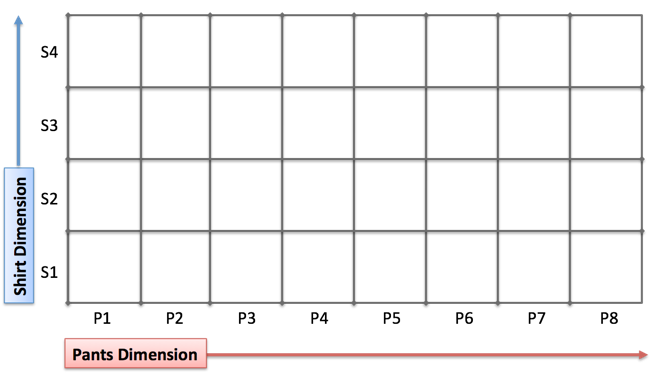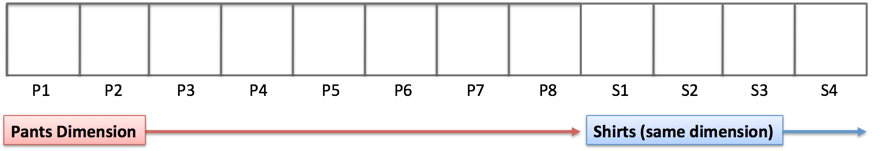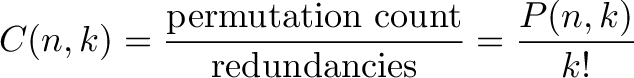When working on a combination problem, we usually multiply. But sometimes addition shows up -- how do we know which is which?

Here's a few mental models I use to keep them straight.

## Mental Model: Different Dimensions

Let's take a simple situation: You have 4 shirts and 8 pants, how many outfits can you make?

In essence, you are picking a spot on this grid:Shirts and Pants exist in separate dimensions, whose area represents distinct solutions. We can pick any spot in the grid and we have 4 x 8 = 32 options.

Now, suppose we had 4 shirts and 8 pants and had to pick a single item to sell. Here, they lie along the same "clothing item" dimension:We can randomly pick any point along the line and have 4 + 8 = 12 options.

Think "different dimensions vs. same dimesion" or "grid vs. line".

## Mental Model: AND vs OR

Another interpretation is AND (multiplication) vs. OR (addition).

Let's say we must pick one of 4 shirts AND one of 8 pants. We need both to stay out of trouble. The scenario is:

pick among 4 shirts AND among 8 pants = 4 * 8 = 32 choices

What if McDonald's softens their regulations and allows a shirt OR pants? (But not both -- yikes.) Then, we have:

pick among 4 shirts OR among 8 pants = 4 + 8 = 12 choices

Writing out the scenario is often easier to think through, especially with numerous dimensions (shirts, pants, hats, shoes).

As you internalize the analogies, you'll quickly recognize whether multiplication or addition is needed.

## Example: Combination & Permutation Formula

Let's go meta for a minute. The permutation formula is:$\displaystyle{ P(n,k) = \frac{n!}{(n-k)!} }$

The numerator ($n!$) is the max volume assuming each of the $n$ choices has its own dimension. The number of rearrangements of 8 people is 8 * 7 * 6 * 5 * 4 * 3 * 2 * 1.

But suppose we only care about the first 3 decisions -- picking a Gold, Silver and Bronze among 8 contestants. In this case, we shrink our solution space by dividing out the 5 dimensions we aren't using (which has 5! options on its own). We are left with 8! / 5! = 8 * 7 * 6 = 336 choices, with the general formula $\frac{n!}{(n-k)!}$.

(If multiplication creates dimensions, then division should remove them.)

Now, let's say the medals are identical: we're giving a tin can to 3 out of 8 people. We need to further remove dimensions, because we have 3! = 3 * 2 * 1 = 6 redundancies for each permutation in our solution space. We again shrink our solution space:$\displaystyle{C(n,k) = \frac{\text{permutation count}}{\text{redundancies}} = \frac{P(n,k)}{k!} }$

(I imagine the solution space volume getting denser.)

Ah! That's what's happening with the combination and permutation formula. We create the max volume and shrink it by the dimensions we are not using. Mentally translate the scenario into a version that makes sense to you.

## Example: Flipping Coins

Here's how I think through a few sample problems.

You flip a coin 10 times. How many ways can you get at least 7 heads?

First off, the total number of possibilities is 2^10 = 1024. Intuitively, I see each flip as a decision along a different dimension, not the same number line. This means we have 2 * 2 * 2 *... possibilities, not 2 + 2+ 2 + ... possibilities.

Geometrically, this would be a 10-dimensional "choice space", or, written out:

(Heads OR tails) AND (Heads OR tails) AND (Heads OR tails) AND ...

Ok. Now, how can we get at least 7 heads? That means we had 0 tails [10 heads], 1 tails [9 heads], 2 tails [8 heads], or 3 tails [7 heads].

Switching to the written description, this becomes:

choices we want = (0 tails OR 1 tail OR 2 tails OR 3 tails)

Given our 10 flips, the number of outcomes are:

• 0 tails = 1 choice (all heads)
• 1 tail = 10 choices (exactly one flip was tails)
• 2 tails = C(10,2) = 10*9/(2*1) = 45 choices based on the combination formula
• 3 tails = C(10,3) = 10 * 9 * 8 / (3 * 2 * 1) = 720 / 6 = 120 choices

So, the total is

choices we want = (1 + 10 + 45 + 120) = 176

And for kicks, the chance of seeing this happen is:

176 / 1024 = 17.2%

Multiplication goes beyond "repeated addition". It's a general notion of combining for which I'm still discovering interpretations. Let's not get tied into a single meaning.

Happy math.

## Appendix: Computer Programming

Turning AND/OR statements into arithmetic maps nicely to Boolean logic.

If A and B are variables with the values 1 or 0, we can write:

• A AND B = A * B
• A OR B = A + B

In most languages, a positive number evaluates to "true", so A + B = 2 is true. Note that this OR is an "inclusive OR" that allows both values to be true. To force an exclusive OR, we could take the remainder after dividing by two:

• A XOR B = (A + B) % 2

Most programming languages have separate operators for AND (&&), OR (||) and XOR (^), but it's nice seeing how logic works with regular arithmetic.

Additionally, "if/then/else" statements can be converted to arithmetic.

If y is a variable (1 or 0) that determines a result, instead of:

if (y) {
result = ResultIfTrue;
}
else {
result = ResultIfFalse;
}


we can use the single statement:

result = y * ResultIfTrue + (1 - y) * ResultIfFalse

This version avoids the needs for branching, which is expensive for a CPU, and is a formula we can optimize with Calculus (used in machine learning algorithms).